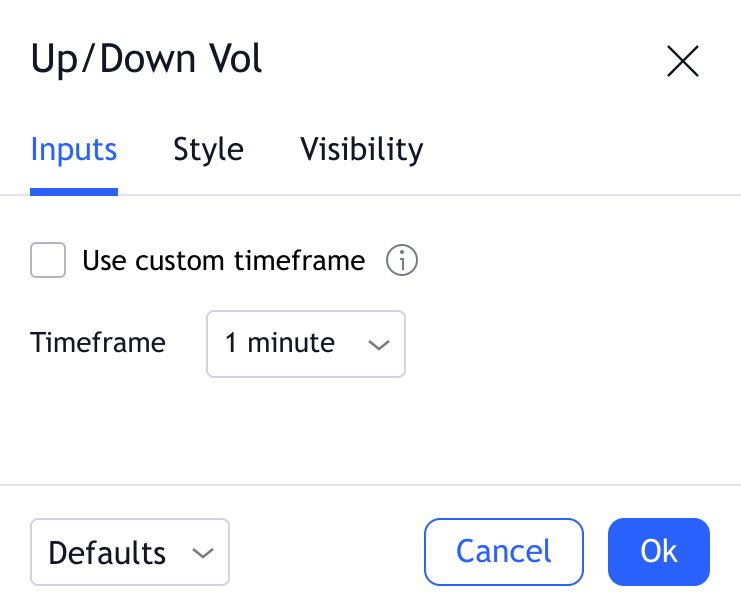# Up/Down Volume

#### Definition

The Up/Down Volume indicator reflects the direction in which the trades were moving within each particular bar. To do this, it splits the volume values on the current bar into two: Up Volume (volume calculated when the price went up) and Down Volume (volume calculated when the price went down). If the Up Volume is greater than the Down Volume, then the main trading volume within the current candle occurred at the purchase price.

The resulting values are plotted as a histogram: Up Volume values are plotted above zero, Down Volume values below zero. The difference between the two values are indicated by Delta, displayed as a notch in the color of the prevailing volume.

#### Calculation

To calculate the Up and Down Volume values, the indicator scans the volume from the candles of a smaller timeframe that are included in the current candle and analyzes in which direction the price moved when these candles formed.

To do this, it first determines the timeframe from which the volume values will be taken. A higher timeframe will provide more historical data, but the calculations will be less accurate.

Different lower timeframes can be used to calculate the Up/Down Volume. By default, the timeframe for the calculation is selected automatically, based on the timeframe of the main chart:

 Chart timeframe Timeframe used for the calculation Intraday 1 Daily 5 Others 60

Indicator values cannot be calculated for timeframe bars below 1 minute.

After the timeframe is selected, each bar of a smaller timeframe is analyzed for the direction in which the price moved.

If the opening price is not equal to the closing price:

• If the closing price of the current bar is higher than its opening price, it is considered that the price was moving up.
• If the closing price of the current bar is less than its opening price, the price is considered to have moved down.

If the closing price of the current bar is equal to the opening price:

• If the closing price of the current bar is greater than or equal to the closing price of the previous one, it is considered that the price was moving up.
• If the closing price of the current bar is less than the closing price of the previous one, it is considered that the price was moving down.

After that, the volume values on all bars where the price moved in the same direction are summed up. Thus, Up Volume shows how much volume was traded when the price was moving up, and Down Volume indicates for trades while movement down.

#### Inputs##### Use custom timeframe

Determines whether the lower timeframe used for the calculation is selected automatically (by default) or manually. If checked, the timeframe will be chosen based on the Timeframe input instead.

##### Timeframe

Allows you to specify a specific timeframe that the indicator will check to look for bars to use while calculating the sum of volumes. Higher timeframes provide more historical data, but the calculation becomes less precise. This field is only used when the Use custom timeframe option is enabled.